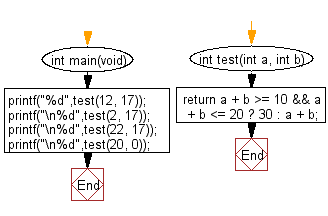﻿ C : Sum 2 integers, if it is in the range 10..20, return 30# C Exercises: Compute the sum of the two given integers. If the sum is in the range 10..20 inclusive return 30

## C-programming basic algorithm: Exercise-17 with Solution

Write a C program to compute the sum of the two given integers. If the sum is in the range 10..20 inclusive return 30.

C Code:

``````#include <stdio.h>
#include <stdlib.h>

int main(void){
printf("%d",test(12, 17));
printf("\n%d",test(2, 17));
printf("\n%d",test(22, 17));
printf("\n%d",test(20, 0));
}

int test(int a, int b)
{
return a + b >= 10 && a + b <= 20 ? 30 : a + b;
}
``````

Sample Output:

```29
30
39
30
```

Pictorial Presentation:Flowchart:C Programming Code Editor:

What is the difficulty level of this exercise?

Test your Programming skills with w3resource's quiz.

﻿

## C Programming: Tips of the Day

Why doesn't a+++++b work?

printf("%d",a+++++b); is interpreted as (a++)++ + b according to the Maximal Munch Rule!.

++ (postfix) doesn't evaluate to an lvalue but it requires its operand to be an lvalue.

! 6.4/4 says the next preprocessing token is the longest sequence of characters that could constitute a preprocessing token"

Ref : https://bit.ly/3fdldUT